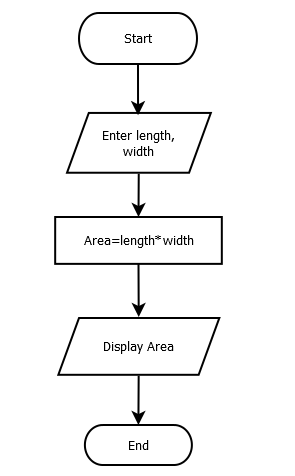# Write a Program to Find Area of Rectangle

Area of a rectangle can be found by multiplying its length and width. You can refer Algorithm and flowchart of this program as well for better understanding.

#### Flowchart:#### Algorithm:

Begin:

Step1: Take two numeric values (length and width) from the user

Step2: Multiply both values and store result into a new variable

Step3: Display the result

End:

#### Program Example

This program is tested and executed using Atom IDE with Python 3.8 version. To find area, we cast user input to float because input function returns string type value.

l=float(input('Enter height of rectangle'))
b=float(input('Enter width of rectangle'))
area = l*b
print("Area = ",area)
Output:
Enter length of rectangle 10
Enter width of rectangle 20
Area = 200.0
Trending